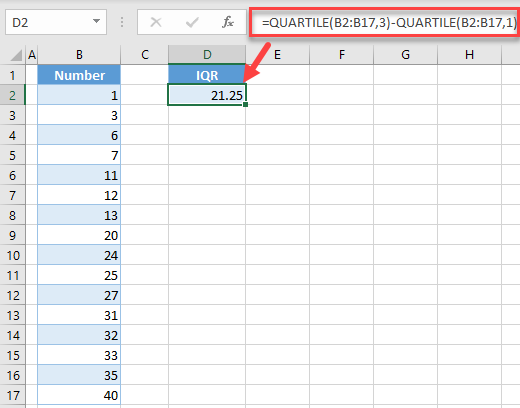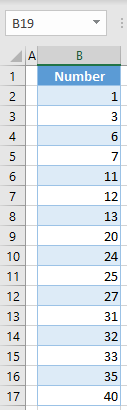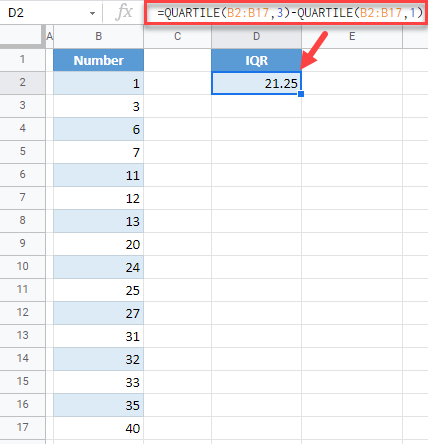# Calculate Interquartile Range (IQR) – Excel & Google Sheets

This tutorial demonstrates how to calculate interquartile range (IQR) in Excel and Google Sheets.## What is Interquartile Range (IQR)?

The Interquartile range presents a spread of the middle half of the dataset. It is actually the difference between the third and the first quartile. Quartiles are values that split a dataset into 4 equal parts. Say. that you have the following dataset:

1, 3, 6, 7, 11, 12, 13, 20, 24, 25, 27, 31, 32, 33, 35, 40

In this case, there are 16 values, so quartiles will split it into 4 parts, each containing 4 numbers. Therefore, the first quartile is 10, the second is 22, and the third is 31.25, From this, you can conclude that the IQR is 31.5-9 = 22.5.

## Calculate Interquartile Range (IQR) in Excel

Say that you have the same dataset in Excel and want to calculate IQR.To achieve this, you need to use the QUARTILE Function to get the first and third quartile. Enter the following formula in cell D2:

``=QUARTILE(B2:B17,3)-QUARTILE(B2:B17,1)``As you can see the IQR is 21.25

## Calculate Interquartile Range (IQR) in Google Sheets

Similar to Excel, to calculate interquartile range in Google Sheets, enter the formula:

``=QUARTILE(B2:B17,3)-QUARTILE(B2:B17,1)``